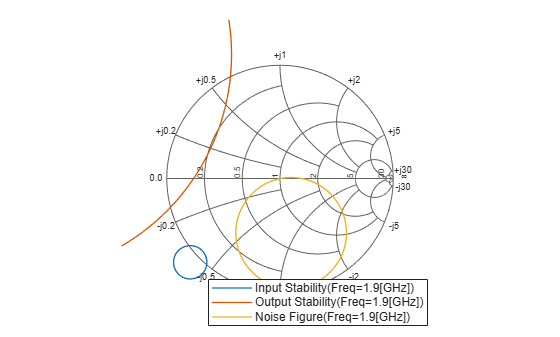# circle

Draw circles on Smith Chart

## Syntax

``[hsm_out] = circle(rfcktobject,freq,type1,value1,....,typen,valuen,hsm1_out)``
``[hlines,hsm] = circle(rfcktobject,freq,type1,value1,....,typen,valuen,hsm_out)``

## Description

example

````[hsm_out] = circle(rfcktobject,freq,type1,value1,....,typen,valuen,hsm1_out)` draws the specified circles on a Smith chart created using the `smithplot` function. The syntax returns an existing `smithplot` handle. ```
````[hlines,hsm] = circle(rfcktobject,freq,type1,value1,....,typen,valuen,hsm_out)` draws the specified circles on a Smith chart. This syntax returns vector handles of line objects and handles of the Smith chart.```

## Examples

collapse all

Create an amplifier object from `default.s2p`.

`amp = read(rfckt.amplifier,'default.s2p');`

Plot the noise figure of the amplifier 1.9 GHz using a Smith chart created using `smithplot` function

```fc = 1.9e9; h = smithplot```
```h = smithplot with properties: Data: [] Frequency: [] Show all properties, methods ```
```circle(amp,fc,'Stab','In','Stab','Out','NF',10.396,h); legend('Location','SouthEast')```## Input Arguments

collapse all

RF Toolbox rfckt object, specified as an object handle,

Single frequency point of interest, specified as a scalar in Hz.

Data Types: `double`

Type value pairs specifying circles to plots, specified as a character vector or a string scalar.

The following table lists the supported circle type options:

`type`Definition
`'Ga'`Constant available power gain circle
`'Gp'`Constant operating power gain circle
`'Stab'`Stability circle
`'NF'`Constant noise figure circle
`'R'`Constant resistance circle
`'X'`Constant reactance circle
`'G'`Constant conductance circle
`'B'`Constant susceptance circle
`'Gamma'`Constant reflection magnitude circle

The following table lists the circle value options:

`value`Definition
`'Ga'`Scalar or vector of gains in dB
`'Gp'`Scalar or vector of gains in dB
`'Stab'``'in'` or `'source'` for input/source stability circle; `'out'` or `'load'` for output/load stability circle
`'NF'`Scalar or vector of noise figures in dB
`'R'`Scalar or vector of normalized resistance
`'X'`Scalar or vector of normalized reactance
`'G'`Scalar or vector of normalized conductance
`'B'`Scalar or vector of normalized susceptance
`'Gamma'`Scalar or vector of non-negative reflection magnitude

Data Types: `char` | `string`

Existing Smith chart handle created using `smithplot` function, specified as an object handle. You can obtain the object handle using ```hsm1 = smithplot('gco')```.

Existing Smith chart handle, specified as an object handle.

## Output Arguments

collapse all

Line objects for circle specifications, returned as a vector of line handles.

Smith chart, returned as an object handle.

Smith chart created using `smithplot` function, returned as an object handle.

## Version History

Introduced in R2007b